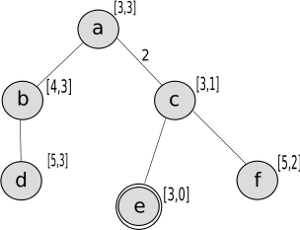Practice Exercise 3.C
Heuristic Search

## 2: Learning Goals

• Define what a heuristic is. Define an admissible heuristic.
• Construct admissible heuristics for appropriate problems. Verify heuristic dominance. Combine admissible heuristics.
• With / Without Costs
• Informed / Uninformed

## 3: Directed Questions

1. What is the distinction between informed and uninformed search? [solution]

2. What is a heuristic? [solution]

3. When is a heuristic admissible? [solution]

4. A* can be seen as a combination of what two search strategies? [solution]

## 4: Exercise: Heuristic Search

1. Consider the search problem represented in the following figure, where a is the start node and e is the goal node. The pair [f, h] at each node indicates the value of the f and h functions for the path ending at that node. Given this information, what is the cost of each arc? The cost <a,c> = 2 is given as a hint. [solution]2. Is the heuristic function h admissible? Explain why or why not. [solution]

3. Trace A* on this problem. Show what paths are in the frontier at each step. [solution]

## 5: Learning Goals Revisited

• Define what a heuristic is. Define an admissible heuristic.
• Construct admissible heuristics for appropriate problems. Verify heuristic dominance. Combine admissible heuristics.